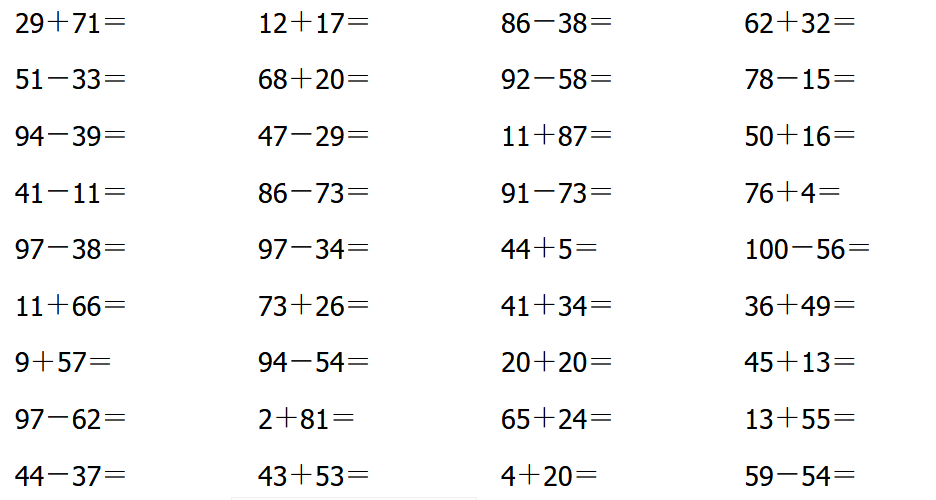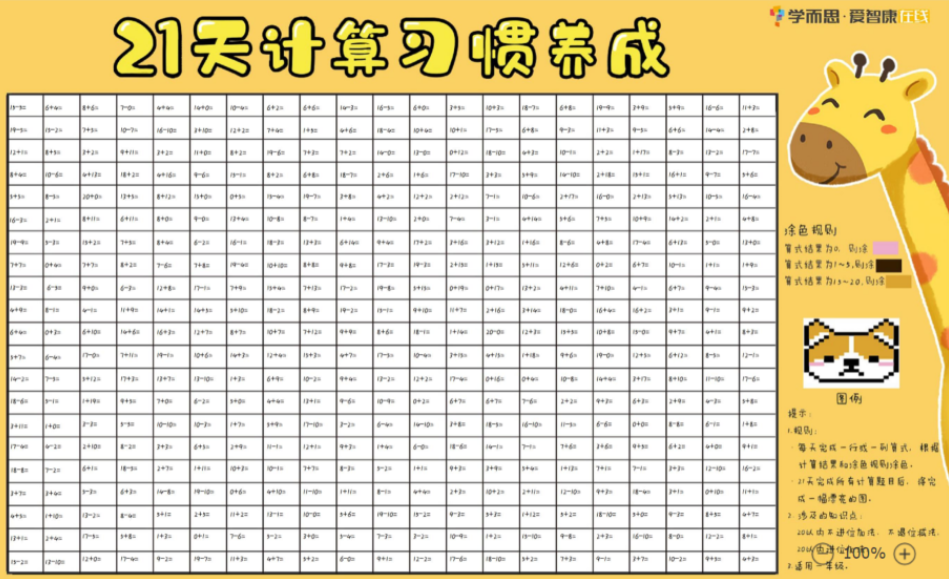# 小学一年级数学题的计算方法

2019-11-25 18:55:56 　来源：网络整理福利资料包大放送_《21天计算习惯养成》大海报pdf版免费领取（分一年级/二年级版）另外学而思爱智康的老师还为大家精心整理了：

《21天计算习惯养成》大海报pdf版

助你效率翻倍！取得优异成绩！

部分资料截图如下：1、正方形 (C：周长 S：面积 a：边长 )

周长=边长×4 C=4a 面积=边长×边长 S=a×a

2、正方体 (V:体积 a:棱长 )

表面积=棱长×棱长×6 S表=a×a×6 体积=棱长×棱长×棱长 V=a×a×a

3、长方形( C：周长 S：面积 a：边长 )

周长=(长+宽)×2 C=2(a+b) 面积=长×宽 S=ab

4、长方体 (V:体积 s:面积 a:长 b: 宽 h:高)

(1)表面积(长×宽+长×高+宽×高)×2 S=2(ab+ah+bh) (2)体积=长×宽×高 V=abh

5、三角形 (s：面积 a：底 h：高)

面积=底×高÷2 s=ah÷2 三角形高=面积 ×2÷底 三角形底=面积 ×2÷高

6、平行四边形 (s：面积 a：底 h：高)

面积=底×高 s=ah

7、梯形 (s：面积 a：上底 b：下底 h：高)

面积=(上底+下底)×高÷2 s=(a+b)× h÷2

8、圆形 (S：面积 C：周长 л d=直径 r=半径)

(1)周长=直径×л=2×л×半径 C=лd=2лr (2)面积=半径×半径×л

9、圆柱体 (v:体积 h:高 s：底面积 r:底面半径 c:底面周长)

(1)侧面积=底面周长×高=ch(2лr或лd) (2)表面积=侧面积+底面积×2

(3)体积=底面积×高 (4)体积=侧面积÷2×半径

10、圆锥体 (v:体积 h:高 s：底面积 r:底面半径)

体积=底面积×高÷3

11、总数÷总份数=平均数

12、和差问题的公式：(和+差)÷2=大数 (和-差)÷2=小数

13、和倍问题： 和÷(倍数-1)=小数 小数×倍数=大数 (或者 和-小数=大数)

14、差倍问题： 差÷(倍数-1)=小数 小数×倍数=大数 (或 小数+差=大数)

15、相遇问题

相遇路程=速度和×相遇时间; 相遇时间=相遇路程÷速度和; 速度和=相遇路程÷相遇时间

16、浓度问题

溶质的重量+溶剂的重量=溶液的重量 溶质的重量÷溶液的重量×100%=浓度

溶液的重量×浓度=溶质的重量 溶质的重量÷浓度=溶液的重量

17、利润与折扣问题

利润=售出价-成本; 利润率=利润÷成本×100%=(售出价÷成本-1)×100%

涨跌金额=本金×涨跌百分比; 利息=本金×利率×时间; 税后利息=本金×利率×时间×(1-20%)QQ扫一扫您将获得群内福利家长互动海量资料同步免费视频课程了解最新信息及时解读资讯你可能感兴趣的文章立即咨询1对1在线课程*学而思爱智康会在24小时内与您取得电话联系大家都在看热门课程介绍限时免费领取学习相关10月13日初中入学规划KET/PET备考

• 全国课程在线咨询
• 咨询热线：4000-121-121
• 扫描注册有礼

• 让学习更有效